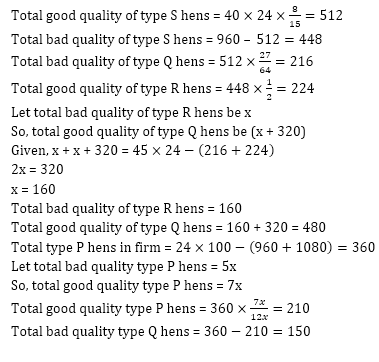# IBPS RRB PO/Clerk Mains Quantitative Aptitude Quiz: 22nd August 2019IBPS RRB PO/Clerk Quantitative Quiz
Do you follow a proper strategy or Study Plan for IBPS RRB Mains 2019? Are you aiming IBPS RRB 2019 this time? If yes, then this is the section which can help you to do wonders if practiced well. A good attempt with a mix of accuracy can help you fetch good marks. The reasoning is a game of wits and mind. It is all about logics that a question may have. Speed and accuracy are what that matters the most in this section. The only way to achieve an ambitious goal is by practicing only. So, attempt the quiz of Reasoning ability that inculcates the important questions from the important topics. Do not miss out to practice the Quantitative aptitude quiz that is being provided on bankersadda.

Direction (1 – 5): Read the direction carefully and answer the questions give below.

Maanik opened a ‘Hen reproductive’ firm in 2017 and in his firm,  there are four types of hens , i.e. P, Q,  R & S. Out of total hens some are good quality & some are bad quality. 40% of total hens in firm are S types and total type Q & type R hens together in firm are 12.5% more than total type S hens in the firm. Total type S hens in firm are 960 and bad quality type S hens in firm are 12.5 % less than total good quality same type of hens, while ratio of total good quality of type S hens in firm to total bad quality of type Q hens in firm is 64 : 27. Total good quality of type R hens in firm are 50% of total bad quality of type S hens and total bad quality of type R hens are 320 less than total good quality of type Q hens in firm. Total good quality of type P hens in firm are 40% more than that of total bad quality of type P hens.

Q1.  Total bad quality type S hens are what
percent more or less than total good quality of type Q hens?
Q2.  Find difference between average number of good quality type P & type R hens and average number of bad quality type Q & type S hens?

(a) 115
(b) 125
(c) 130
(d) 135
(e) 145
S2. Ans(a)
Sol.
S5. Ans(e)
Sol.Q6. Suresh, Aman and Vikram are partners in a business. Suresh invested Rs. 4000 for whole year. Aman invested Rs. 6000 initially but increased this investment up to Rs. 8000 at the end of 4 months, while Vikram invested Rs, 8000 initially, but withdraw Rs. 2000 at the end of 9 months. At the end of year total earned profit is Rs. 16950, find their share of profit.
(a) Rs 3600, Rs 6600, Rs 6750
(b) Rs 2000, Rs 3050, Rs 5400
(c) Rs 2450, Rs 2460, Rs 1456
(d) Rs 2400, Rs 2480, Rs 1406
(e) None of these

Q7. A circus tent is cylindrical up to a height of 3 m and conical above it. If its diameter is 105m and the slant height of the conical part is 63 m, then the total area of the canvas required to make the tent is (take π=2/7)
(a) 11385 m2
(b) 10395 m2
(c) 9900 m2
(d) 990 m2
(e) 10435 m2

S7. Ans.(a)
Sol.
Q8. Amit sold an article for Rs. 15000. Had he offered a discount of 10% on the selling price, he would have earned a profit of 8%. What is the cost price ?
(a) Rs. 12500
(b) Rs. 13500
(c) Rs. 12250
(d) Rs. 13250
(e) Rs. 10350
Q9. A tank is filled in 5 hours by three pipes P, Q and R The pipe R is twice as fast as Q and Q is twice as fast as P. How much time will pipe P alone take to fill the tank?
(a) 35 hours
(b) 25 hours
(c) 20 hours
(d) Cannot be determined
(e) None of these
S9. Ans.(a)
Sol.Q10. Rs. 2,000 is invested at 10% p.a. on simple interest. If that interest is added to the principal after every 20 years, the amount will become Rs. 14,000 after
(a) 15 years
(b) 331/3 years
(c) 162/3 years
(d) 40 years
(e)None of these

Q11.  66.99% of 1799.98 + (?)2 +  6.98 % of  599.99 = (37.98)2
(a) 10
(b) 14
(c) 18
(d) 22
(e) 26

S11. Ans(b)
Sol.
(c) 336
(d) 252
(e) 224
S12. Ans(d)
Sol.
(a) 12
(b) 16
(c) 19
(d) 25
(e) 29
S13. Ans(a)
Sol.

Q14. ?/47.98+ 25.98 % of 249.89 – 19.99% of 74.98 = (7.99)2
(a) 864
(b) 816
(c) 768
(d) 720
(e) 672

S14. Ans(e)
Sol.